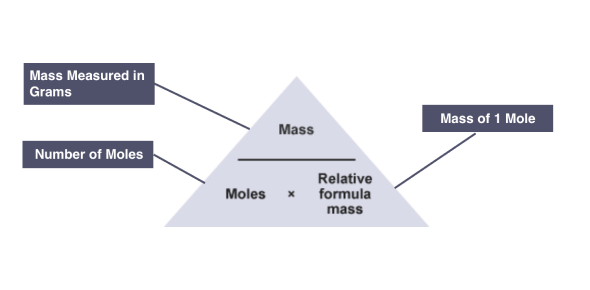# Calculation In Chemistry IGCSE Quiz!

8 Questions | Attempts: 729SettingsAre you familiar with calculations in chemistry, and do you think you can pass this quiz? In a chemical reaction, one or more reactants are converted into products. The objective of a chemical equation is to express this relation in terms of the actual reactants' formulas and items that identify a specific chemical change. If you are interested in how calculation in chemistry works, this is the quiz for you.

• 1.
The formula of Iron III hydroxide is:
• A.

Fe(OH)3

• B.

Fe(OH)2

• C.

Fe(OH)4

• D.

FeOH

• 2.
The relative formula mass of Calcium hydroxide Ca(OH)2 is:
• A.

74g

• B.

57g

• C.

74amu

• D.

57amu

• 3.
The mass of 2 mol of carbon dioxide CO2 is:
• A.

88 amu

• B.

44g

• C.

88 g

• D.

44 amu

• 4.
How many moles are there in 9.8g of sulphuric acid H2SO4?
• A.

1 mol

• B.

2 mol

• C.

0.1 mol

• D.

0.2 mol

• 5.
The number of atoms found in one mole of silver atoms is called?
• A.

Wissam's number

• B.

• C.

Newton's number

• D.

Pascal's number

• 6.
When the following equation is balanced, the sum of all coefficients will be   H2  +  O2  --->   H2O
• A.

2

• B.

3

• C.

4

• D.

5

• 7.
When the following chemical equation is balanced, the coefficients will be respectively   N2  +  H2  -->  NH3
• A.

1 - 2 - 3

• B.

3 - 2 - 1

• C.

1 - 3 - 2

• D.

0 - 3 - 2

• 8.
5 moles of C6H12O6 has a mass:
• A.

90

• B.

900

• C.

9

• D.

0.9

## Related TopicsBack to top
×

Wait!
Here's an interesting quiz for you.